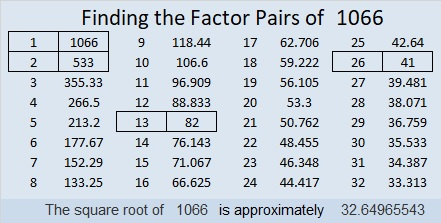# 1066 and Level 4

Some of the clues in today’s puzzle were used in previous puzzles this week. Sometimes their factors have to be exactly the same as they were in the previous puzzles, but sometimes they might not be. Can you figure out where to put the numbers 1 to 12 in both the first column and the top row so that those numbers are the factors of the clues given?Print the puzzles or type the solution in this excel file: 12 factors 1063-1072

Here are a few facts about the number 1066:

• 1066 is a composite number.
• Prime factorization: 1066 = 2 × 13 × 41
• The exponents in the prime factorization are 1, 1, and 1. Adding one to each and multiplying we get (1 + 1)(1 + 1)(1 + 1) = 2 × 2 × 2 = 8. Therefore 1066 has exactly 8 factors.
• Factors of 1066: 1, 2, 13, 26, 41, 82, 533, 1066
• Factor pairs: 1066 = 1 × 1066, 2 × 533, 13 × 82, or 26 × 41
• 1066 has no square factors that allow its square root to be simplified. √1066 ≈ 32.64965529² + 15² = 1066
25² + 21² = 1066

1066 is the hypotenuse of FOUR Pythagorean triples:
616-870-1066 is 2 times (308-435-533) and calculated from 29² – 15², 2(29)(15), 29² + 15²
410-984-1066 which is (5-12-13) times 82
234-1040-1066 which is 26 times (9-40-41)
184-1050-1066 is 2 times (92-525-533) and calculated from 25² – 21², 2(25)(21), 25² + 21²

1066 looks interesting when it is written in some other bases:
It’s palindrome 1110111 in BASE 3 because 3⁶ + 3⁵ + 3⁴ + 3² + 3¹ +3⁰ = 1066,
13231 in BASE 5 because 1(5⁴) + 3(5³) + 2(5²) + 3(5) + 1(1) = 1066,
1414 in BASE 9 because 1(9³) + 4(9²) + 1(9) + 4(1) = 1066, and
2I2 in BASE 19 (I is 18 base 10) because 2(19²) + 18(19) + 2(1) = 1066